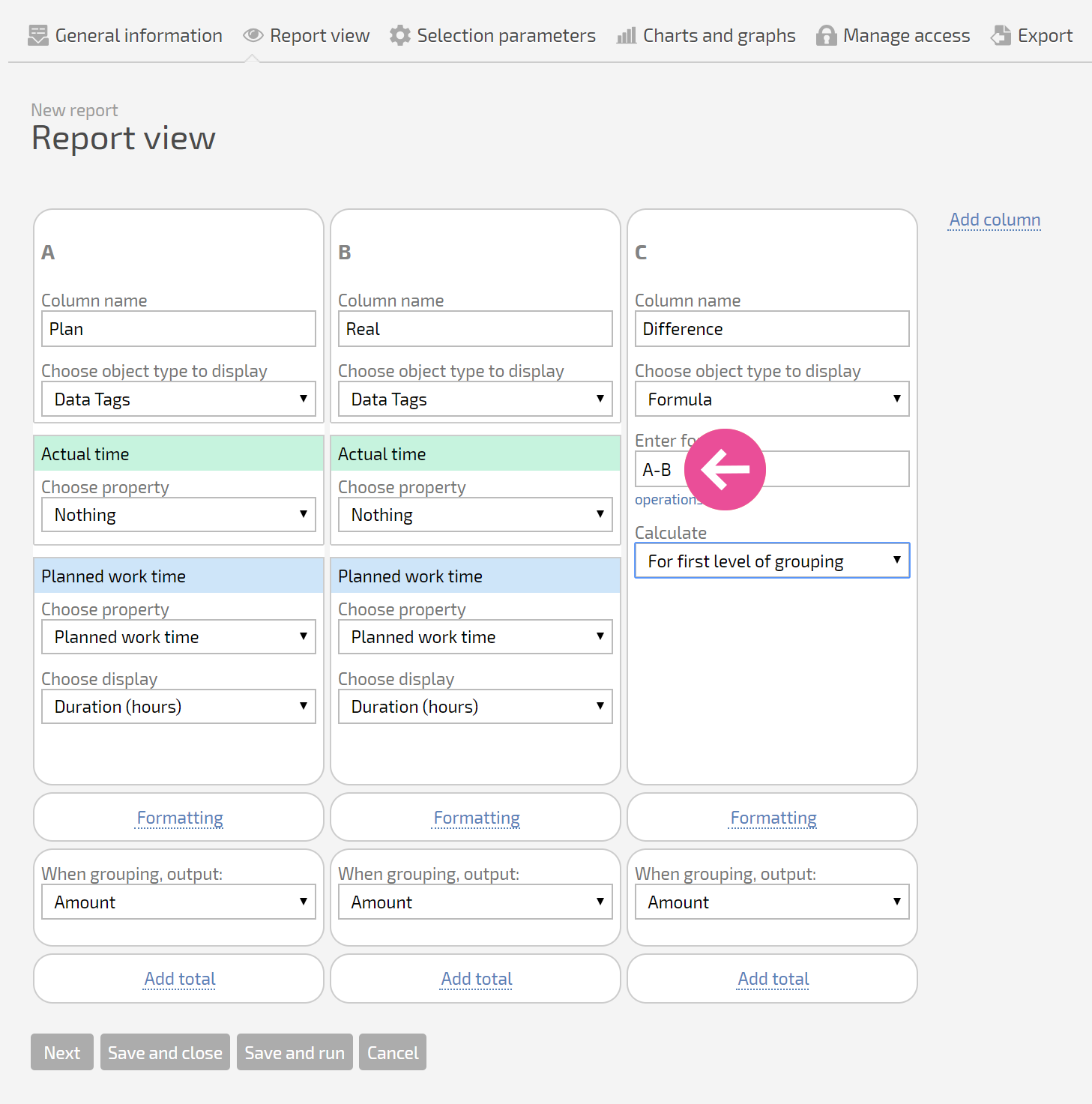# Reports: Formulas

(diff) ← Older revision | Latest revision (diff) | Newer revision → (diff)

Planfix reports let you use simple formulas in columns to calculate values. Formulas use data entered in columns located to the left of the formula column (up to the column with the formula); they use standard operators. The data from the columns is denoted by the letter designated to the column:Formulas are calculated in order from left to right, in one pass: rows are filled with values from the database for all non-formula columns, then formulas are calculated. As a result, formulas normally use values from the database up to the formula column, but they don't use the values of other formulas.

You can use standard operators and Planfix functions in formulas.